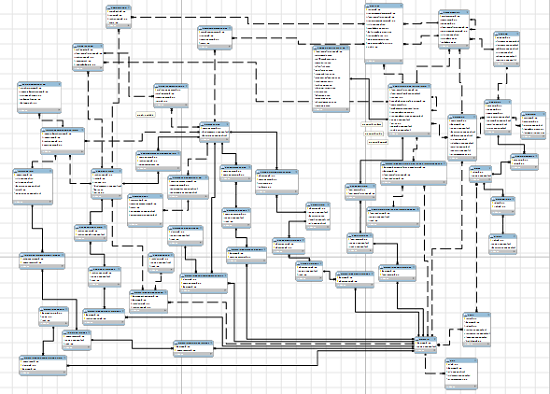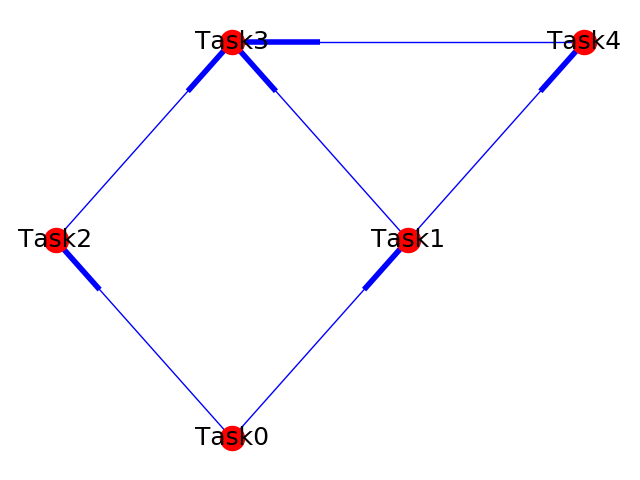Consider the ERD (Entity Relationship Diagram) in the picture above. How does an ETL Developer work out which order to load the tables in to make sure no errors are thrown due to foreign key constraints? This is where DAGs come in very handy.

DAG stands for Directed Acyclic Graph. Let’s start of with the G, Graph; A collection of nodes and edges (which connect the nodes). For most ETL services, a specific job / task would resemble a node and a dependency between any two jobs / tasks would resemble an edge. The D, Directed tells us that an edge has a certain direction associated to it which tells us the direction of the dependency between two nodes. And lastly the A, Acyclic which tells us that there can’t be a cycle or a closed path.

Below you will see a Sample DAG.Each Task represents a node and each fattened line represents an edge (dependency between two nodes)

As we can see from the diagram above:

The sequence of events could be as follows:

I wanted to implement my own mini DAG framework which can be found here: DAG Framework. What I like about this approach is that the dependencies between tasks is encapsulated within each class as opposed to setting all the dependencies at the end of a module which is what I have seen in a number of frameworks.

The above DAG example can be seen in action using the framework and example module provided in the repository.

To run it yourself, simply open up a Python shell from root folder (data-analysis)